# Find the minimum numerical value in a list based on a criteria in Excel 2010

To find the minimum numerical value in a list, based on a criteria, we can use the MIN function along with the If function in Excel 2010.

MIN: This function is used to return the smallest number in a set of values. It ignores logical values and text.

Syntax of “MIN” function: =MIN(number1, [number2],….)

Example: Range A1:A4 contains a list of numbers from which we need to find the minimum number.

• Select the cell B1 and write the formula.
• =MIN(A1:A4), press Enter on your keyboard.
• The function will return 3.
• 3 is the minimum value in the range A1:A4.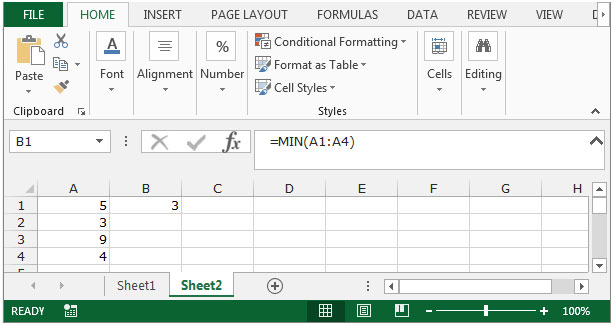IF: - Check whether a condition is met and returns one value if True and another value if False.

The syntax of “IF” function =if(logical test,[value_if_true],[value_if_false])

First the formula will do the logical test, and then return the output based on whether the logical test evaluates to true or false.

For example:Cells A2 and A3 contain the number 3 and 5. If the number is 3, the formula should return “Yes” else “No”.

=IF (A1=3,"Yes","No")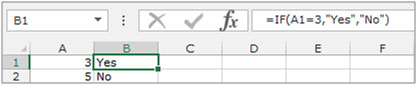Let’s take an example to understand how we can find the minimum numerical value in a list based on certaincriteria.

We have data for certain items in range A2:D24. Column A contains items, Column B contains zone and column C contains sold unit.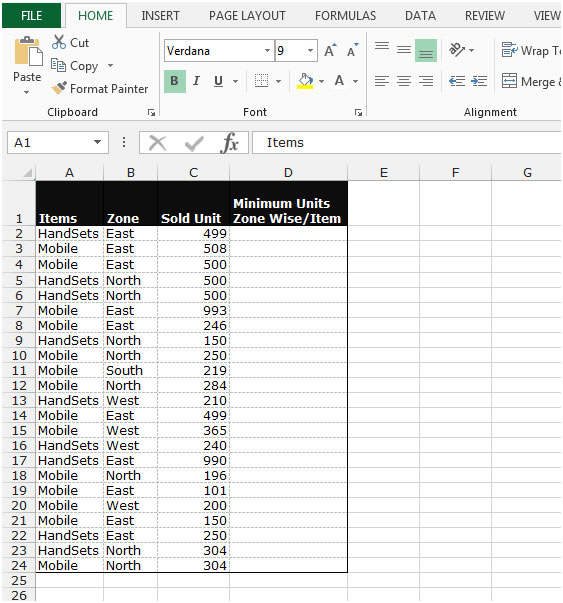We need to find the minimum sold quantity zone wise for each item.

• Select the cell D2.
• Write the formula to find out the minimum value
• =MIN(IF(\$A\$2:\$A\$31=A2,IF(\$B\$2:\$B\$31=B2,\$C\$2:\$C\$31,""),""))
• Press Ctrl+Shift+Enter.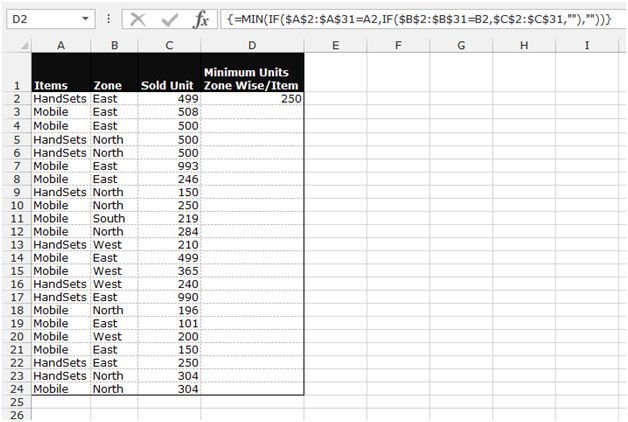• The function will return the minimum value in the east zone for handsets.
• By using this formula, you can find out the minimum value for each zone item-wise.

Note: This is an array function, hence you cannot paste the formula in multiple cells. You will have to paste the formula one by one in each cell.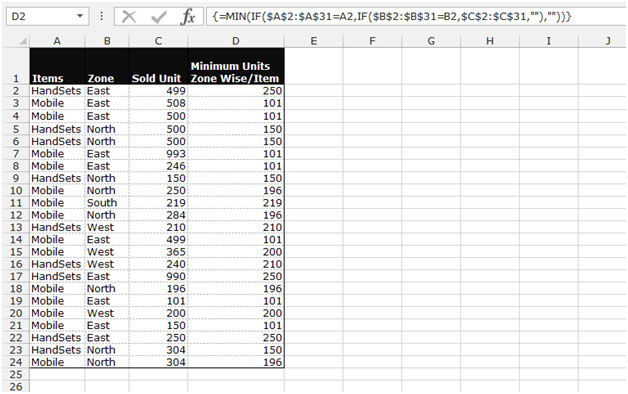This is the way we can find out the minimum number in the list based on certain criteria in Microsoft Excel 2010 and 2013.

1.